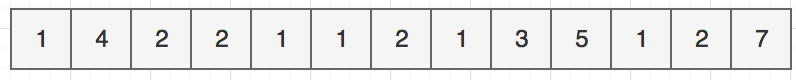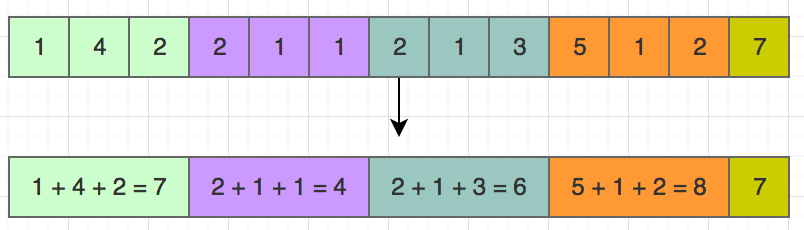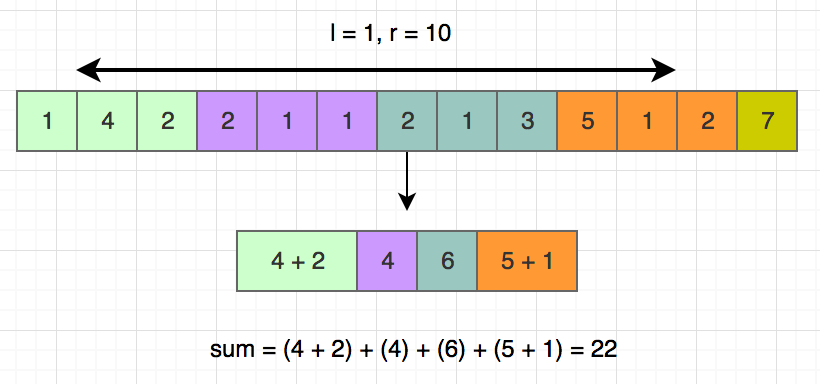# Square Root Decomposition

Using the square-root decomposition technique, we can reduce the time complexity of several types of problems from $O(n)$ to $O(Sqrt(n))$. I will start with an example. Let’s say we have an integer array and we have to perform several operations on them. The operations can be of two types. The first type is, we have the find the sum of all the integers between index $l$ to $r$. The second type is, we have to update the value of index $i$. Some of you may already know how to solve this using a segment tree or binary search tree, today we will solve it with a new technique.What is the naive way to solve this? Every time we are asked to find the sum, we will loop from $l$ to $r$, and for an update operation, we will update the value in index $i$. In this case, the complexity to find the sum is $O(n)$.

Now let’s try to optimize it. We will divide the array into multiple segments. Every segment will contain the sum of all integers of that segment. See the picture below:The size of the array is $13$. When we create the segments, we want to ensure that the size of every part is almost the same. Also, the number of segments in the array should be nearly the same as the size of the segments. That’s where square root helps us. The square root of $13$ is approximately $3.61$ or $3$ if we ignore the decimal points. If we divide the array into segments of size $3$, we will get $13/3 = 4$ segments. The size of each segment will be $3$ except for the last part.

If the size of the array is not a square number, we will get one extra segment which is a bit smaller, but that’s not a problem. We will see soon how dividing in this way will help us immensely.

Now when we are asked to find the sum in the range $[l, r]$, we will check which segments fall into the range, and we will get the sum for those segments.

At first, we need to pre-process the segments. See the code below:

We are traversing the input array and every time $i % segment_size == 0$ becomes true, we go to next segment.

For $[l, r] = [1, 10]$, we need to find sum for the segments shown in the picture below:The problem is, some of the segments are only partially in the range. The first and last segments can have this issue. In that case, we need to loop through each index of those segments. For other segments, we can get the sum. We have $sqrt(n)$ segments, and max size of each segment is also $sqrt(n)$. So even though we may need to loop through the first and the last segment, the total complexity remains $O(sqrt(n))$. Now you know why we divided the array this way.

Now one small question. For a particular index $i$, how do we know which segment $i$ is situated it? Very easy, just check the value of $i/segment_size$.

Let’s see the code for sum operation:

We divided the the code in 3 parts as discussed above.

Now lets come to update operation. It’s very easy, just find the segment $i$ index is located it and update the sum for that segment.

The complexity for update is $O(1)$.

#### Whats next?

You can even find the Lowest Comment ancestor of a tree using a similar technique. If you want the dig deeper, check out this PDF.